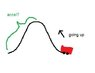# Acceleration of Rollercoaster hill?

• alliereid
In summary, to calculate the acceleration of the train during the trip down the first hill, you can use the equation d=V(initial)(t)+.5(a)(t)^2 and consider the potential energy at the top and the velocity it will have at the bottom of the hill. Using the equation v = a*t, you can find the acceleration of the train.

## Homework Statement

Calculate the acceleration of the train during the trip down the first hill

Time for train to go from top of hill to bottom:3.5 s
Time for train to pass point at top of hill 1.5s
Time for train to go from bottom to top of first hill:7 s
Time for train to pass point of bottom of hill:1s
Height of hill: 16.155 m

## Homework Equations

d=V(initial)(t)+.5(a)(t)^2

## The Attempt at a Solution

I tried using that equation but there is two variables missing distance and acceleration. I can't use the height of the hill because isn't this a x problem (parallel problem)? I don't understand how I can find the acceleration of the rollercoaster train during the trip down the hill with only this data.

With no diagram you need to be more specific.

Okay I attached a diagram. As you can see, the train is going up and I'm trying to find the accel from the top of the hill to the bottom.

#### Attachments

•diagram.jpg
8.2 KB · Views: 490
Until approved no one can see the picture.

But consider the potential energy at the top and the velocity it will have at the bottom of the hill.

m*g*h = 1/2*m*v2

so ...

v2 = 2*g*h

Also you should know that

v = a*t

Looks like you may have enough info after all.